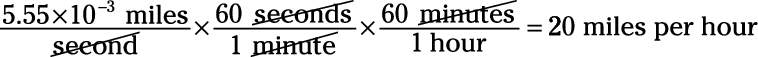##### Physics I Workbook For Dummies with Online Practice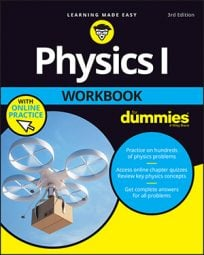In physics terms, acceleration is the amount by which your velocity changes in a given amount of time. In terms of equations, it works like this: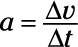Given initial and final velocities, vi and vf, and initial and final times over which your speed changed, ti and tf, you can also write the equation like this: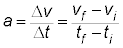To get the units of acceleration, you divide velocity by time as follows: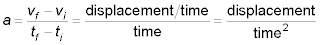Displacement over time squared? Don’t let that throw you. You end up with time squared in the denominator just because it’s velocity divided by time — that’s something you get used to when solving physics problems. In other words, acceleration is the rate at which your velocity or speed changes because rates have time in the denominator.

So for acceleration, you can expect to see units of meters per second2, or centimeters per second2, or miles per second2, or feet per second2, or even kilometers per hour2. Remember that meters per second2 really just means meters per second per second. You can also have units of acceleration such as miles per hour per second or kilometers per hour per minute.

Like velocity, acceleration has magnitude and direction. For example, a car might be traveling at +25 m/s north as it reaches a red stop light. An acceleration of –5 m/s2 (that is, an acceleration toward the south) may bring the car safely to a stop at the light. An acceleration of +5 m/s2 will likely result in an accident!

## Sample question

1. Suppose you’re driving 90.0 kilometers an hour and suddenly see red flashing lights in the rearview mirror. “Great,” you think, and you pull over, taking 20.0 seconds to come to a stop. What was your average acceleration, in m/sec2?

–1.25 m/sec2

1. First convert to kilometers per second: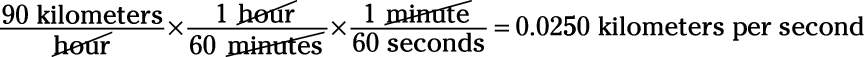2. Convert from kilometers per second to meters per second: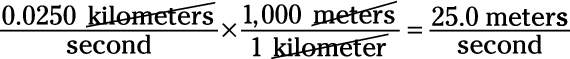3. 3.What was your acceleration? That calculation looks like this: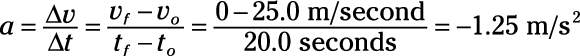In other words, –1.25 m/s2, not +1.25 m/s2. There’s a big difference between positive and negative in terms of solving physics problems — and in terms of law enforcement. If you accelerated at +1.25 m/s2 instead of accelerating at –1.25 m/s2, you’d end up going 180 kilometers per hour at the end of 20.0 seconds, not 0 kilometers per hour.

In other words, the sign of the acceleration tells you how the velocity is changing. A positive acceleration means the velocity is increasing in the positive direction, and a negative acceleration (also known as deceleration) tells you the velocity is increasing in the negative direction. Note that this is not the same as whether your speed is increasing or decreasing. For example, a car that has a velocity of –25 m/s and an acceleration of –3 m/s2 is increasing in speed.

## Practice questions

1. A rocket ship is going to land on the Moon in exactly 2.0 hours. There’s only one problem: It’s going 17,000 miles an hour.

What does its acceleration need to be, in miles per second2, to land on the moon safely at 0.0 miles per hour?

2. You’re stopped at a red light when you see a monster SUV careening toward you.

In a lightning calculation, you determine you have 0.8 seconds before it hits you and you must be going at least 1.0 miles an hour forward at that time to avoid the SUV. What must your acceleration be, in miles per hour2? Can you avoid the SUV?

3. 3.A bullet comes to rest in a block of wood in 1.0 x 10–2 seconds, with an acceleration of –8.0 x 104 m/s2.

What was its original speed, in meters per second?

4. The light turns red, and you ease to a halt. Checking your stopwatch, you see that you stopped in 4.5 seconds. Your acceleration was 1.23 x 10–3 miles per second2 in a direction opposite to your original velocity.

What was your original speed in miles per hour?

Following are answers to the practice questions:

1. 6.6 x 10–4 miles per second2

1. Start by converting 17,000 miles per hour into miles per second: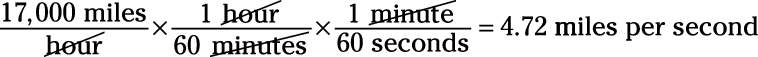2. To land on the moon, vf must be 0 miles per second, and tfto = 2.0 hours, or 2.0 x 3,600 seconds = 7,200 seconds, so: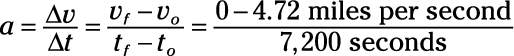3. Calculating this yields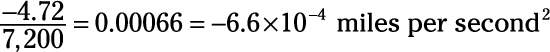So the rocket needs a constant acceleration of 6.6 x 10–4 miles per second2 in a direction opposite to the rocket’s initial velocity to land on the Moon at a speed of 0 miles per second, touching down lightly.

2. 4.5 x 103 miles per hour2. You will avoid the collision.

1. Start by converting 0.8 seconds into hours to get all the quantities in units you want — miles and hours: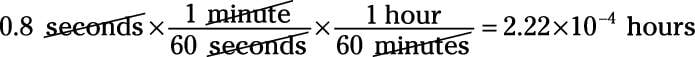2. Calculate the acceleration needed to get you to 1.0 miles per hour: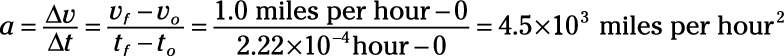3. 800 m/s

1. You can calculate the change in velocity, because it’s acceleration multiplied by time: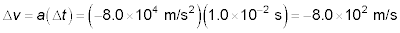2. So if the bullet lost 800 m/s of speed to come to a rest (v = 0), it must have been going 800 m/s originally.

4. 20 miles per hour

1. The change in velocity is acceleration multiplied by time, so: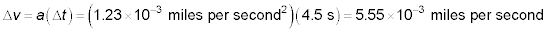2. Convert this result to miles per hour: